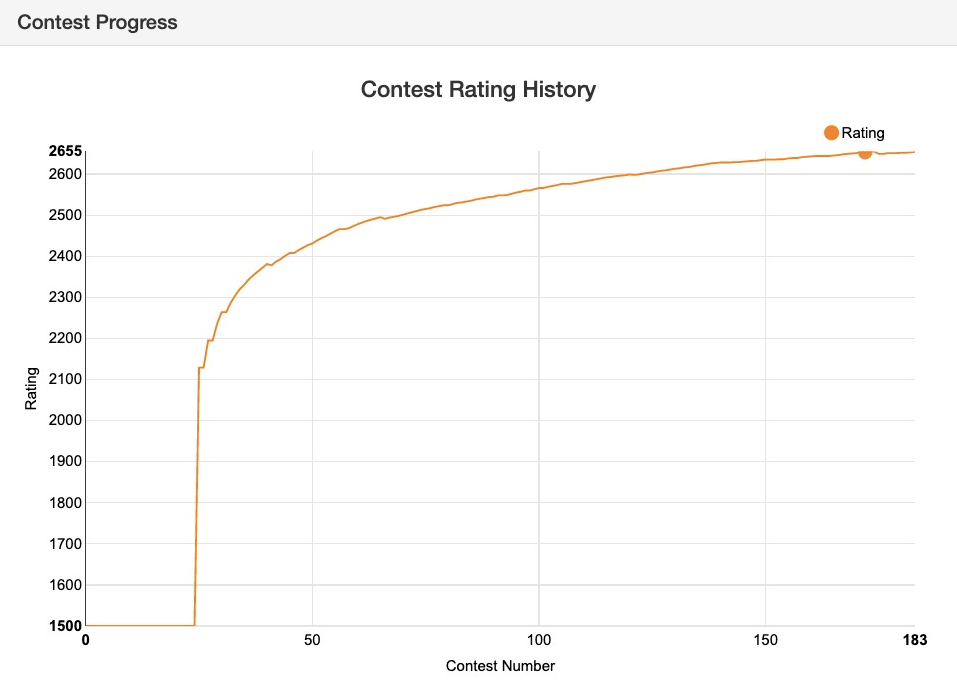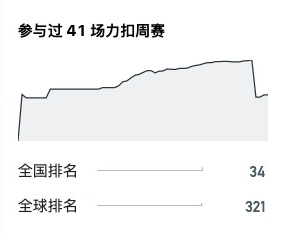📣 全新「周赛评分算法」即将上线，现邀扣友们前来出谋划策！### 0x01 新算法的目标

1. 用户在参加了很多次比赛以后，旧算法的分数更新非常小：1. 旧算法下，用户如果忘记参赛，分数会被扣非常多：1. 在解决问题 1 的同时，分数不能波动太大。

2. 我们会把忘记参赛的人特判出来，不算在参赛者里。但是为了避免 “保分” 的现象，依然需要给忘记参加的用户一些惩罚

### 0x02 ELO 算法介绍

$E_{A, B}=\frac{1}{1+10^{(Rating_B-Rating_A)/400}}$

$Rating^\prime_A=Rating_A+K(S_A-E_{A, B})$

### 0x03 新算法介绍

$ERank_i=\sum^n_{j=1}E_{j, i}+1 (j \neq i)$

$m_i=\sqrt{ERank_i \times Rank_i}$

$\sum^n_{j=1}\frac{1}{1+10^{(Rating_j-ERating_i)/400}}+1=m_i$

$\Delta = f(k) \times (ERating_i - Rating_i)$

$Rating^\prime_i=Rating_i+\Delta$

### 0x06 一些结论

• 从图上可以看出，新的算法可以有效解决分数收敛过快的问题，同时在用户参赛一定场次以后，分数依然会根据排名有可见的波动。

• 经过我们的对比，用新算法得到的前十名的平均竞赛排位比用旧算法得到的更靠前，说明新算法可以更准确地反映选手的算法水平。

• 新算法对最近几场比赛更为看重，在近期的比赛中排名靠前的选手的分数普遍更高。相比旧算法，新算法更具时效性。

👉 戳我

### 0x08 大家来讨论

• 力扣平台非常重视用户的成长。我们观察到有一些用户在一段时间内的水平得到了很大的提升，但由于他们参加了很多场周赛，每次的加分没有真实反映他们的算法水平。所以在这里征询以下大家的意见，我们是否需要赛季？如果需要的话，我们提供两个长度选项：

• 半年

• 一年

• 我们应该如何给忘记参赛的用户惩罚？我们提供两个选项：

• 算分数的时候扣一个固定百分比的分（如 $3%$），e.g. 如果比赛前是 2000 分且忘记参赛了，那么比赛后就是 1940 分。（目前采用的方案）

• 一年（或者一赛季）一次【免死金牌】，忘记参赛也不扣分。第二次及以后的忘记参赛会以输给所有人来计算分数。